# 1 Introduction

## 1.1 Rationale for the package

There has been a long relationship between the disciplines of Epidemiology / Public Health and Biostatistics. Students frequently find introductory textbooks explaining statistical methods and the maths behind them, but how to implement those techniques in a computer is most of the time not explained.

One of the most popular statistical software’s in public health settings is Stata. Stata has the advantage of offering a solid interface with functions that can be accessed via the use of commands or by selecting the proper input in the graphical unit interface (GUI). Furthermore, Stata offers “Grad Plans” to postgraduate students, making it relatively affordable from an economic point of view.

R is a free alternative to Stata. The use of R keeps growing; furthermore, with the relatively high number of packages and textbooks available, it’s popularity is also increasing.

In the case of epidemiology, there are already some good packages available for R, including: Epi, epibasix, epiDisplay, epiR and epitools. The pubh package does not intend to replace any of them, but to only provide a common syntax for the most frequent statistical analysis in epidemiology.

## 1.2 Syntax: the use of formulas

Most students and professionals from the disciplines of Epidemiology and Public Health analyse variables in terms of outcome, exposure and confounders. The following table shows the most common names used in the literature to characterise variables in a cause-effect relationships:

Response variable Explanatory variable(s)
Outcome Exposure and confounders
Outcome Predictors
Dependent variable Independent variable(s)
y x

In R, formulas are used to declare relationships between variables. Formulas are common in classic statistical tests and in regression methods.

Formulas have the following standard syntax:

y ~ x, data = my_data

Where y is the outcome or response variable, x is the exposure or predictor of interest and my_data specifies the name of the data frame where x and y can be found.

The symbol ~ is used in R for formulas. It can be interpreted as depends on. In the most typical scenario, y ~ x means y depends on x or y is a function of x:

y = f(x)

Using epidemiology friendly terms:

Outcome = f(Exposure)

Is worth to mention that Stata requires for variables to be given in the same order as in formulas: outcome first, predictors next.

The pubh package integrates well with other packages of the tidyverse which use formulas and the pipe operator %>% to add layers over functions. In particular, pubh uses ggformula as a graphical interface for plotting and takes advantage of variable labels from sjlabelled. This versatility allows it to interact also with tables from moonBook and effect plots from sjPlot.

### 1.2.1 Stratification

One way to control for confounders is the use of stratification. In Stata stratification is done by including the by option as part of the command. In the ggformula package, one way of doing stratification is with a formula like:

y ~ x|z, data = my_data

Where y is the outcome or response variable, x is the exposure or predictor of interest, z is the stratification variable (a factor) and my_data specifies the name of the data frame where x, y and z can be found.

## 1.3 Contributions of the pubh package

The main contributions of the pubh package to students and professionals from the disciplines of Epidemiology and Public Health are:

1. Use of a common syntax for the most used analysis.
2. A function, glm_coef that displays coefficients from most common regression analysis in a way that can be easy interpreted and used for publications.
3. Integration with the ggformula package, introducing plotting functions with a relatively simple syntax.
4. Integration with the most common epidemiological analysis, using the standard formula syntax explained in the previous section.

# 2 Descriptive statistics

Many options currently exists for displaying descriptive statistics. I recommend the function mytable from the moonBook package for constructing tables of descriptive statistics where results don’t need to be stratified.

In Public Health and Epidemiology it is common to have a categorical outcome and thus, report descriptive statistics stratified by the outcome. Package pubh introduces the function cross_tab as a wrapper to functions from mytable. The idea is to construct these tables, using formulas, in a simple way.

The estat function from the pubh package displays descriptive statistics of continuous outcomes and like mytable, it can use labels to display nice tables. estat. As a way to aid in the understanding of the variability, estat displays also the relative dispersion (coefficient of variation) which is of particular interest for comparing variances between groups (factors).

rm(list = ls())
library(car)
library(broom)
library(mosaic)
library(tidyverse)
library(ggfortify)
library(huxtable)
library(jtools)
library(latex2exp)
library(pubh)
library(sjlabelled)
library(sjPlot)
library(sjmisc)

theme_set(sjPlot::theme_sjplot2(base_size = 10))
theme_update(legend.position = "top")
options('huxtable.knit_print_df' = FALSE)
options('huxtable.autoformat_number_format' = list(numeric = "%5.2f"))
knitr::opts_chunkset(comment = NA) We will use a data set about a study of onchocerciasis in Sierra Leone. data(Oncho) Oncho %>% head() # A tibble: 6 x 7 id mf area agegrp sex mfload lesions <dbl> <fct> <fct> <fct> <fct> <dbl> <fct> 1 1 Infected Savannah 20-39 Female 1 No 2 2 Infected Rainforest 40+ Male 3 No 3 3 Infected Savannah 40+ Female 1 No 4 4 Not-infected Rainforest 20-39 Female 0 No 5 5 Not-infected Savannah 40+ Female 0 No 6 6 Not-infected Rainforest 20-39 Female 0 No  A two-by-two contingency table: Oncho %>% mutate( mf = relevel(mf, ref = "Infected") ) %>% copy_labels(Oncho) %>% cross_tab(mf ~ area) %>% theme_pubh() Infection InfectedNot-infectedTotal (N=822)(N=480)(N=1302) Residence - Savannah 281 (34.2%)267 (55.6%)548 (42.1%) - Rainforest541 (65.8%)213 (44.4%)754 (57.9%) Table with all descriptive statistics except id and mfload: Oncho %>% select(- c(id, mfload)) %>% mutate( mf = relevel(mf, ref = "Infected") ) %>% copy_labels(Oncho) %>% cross_tab(mf ~ area +.) %>% theme_pubh() Infection InfectedNot-infectedTotal (N=822)(N=480)(N=1302) Residence - Savannah 281 (34.2%)267 (55.6%)548 (42.1%) - Rainforest 541 (65.8%)213 (44.4%)754 (57.9%) Age group (years) - 5-9 46 ( 5.6%)156 (32.5%)202 (15.5%) - 10-19 99 (12.0%)119 (24.8%)218 (16.7%) - 20-39 299 (36.4%)125 (26.0%)424 (32.6%) - 40+ 378 (46.0%)80 (16.7%)458 (35.2%) Sex - Male 426 (51.8%)190 (39.6%)616 (47.3%) - Female 396 (48.2%)290 (60.4%)686 (52.7%) Severe eye lesions? - No 640 (77.9%)461 (96.0%)1101 (84.6%) - Yes 182 (22.1%)19 ( 4.0%)201 (15.4%) Next, we use a data set about blood counts of T cells from patients in remission from Hodgkin’s disease or in remission from disseminated malignancies. We generate the new variable Ratio and add labels to the variables. data(Hodgkin) Hodgkin <- Hodgkin %>% mutate(Ratio = CD4/CD8) %>% var_labels( Ratio = "CD4+ / CD8+ T-cells ratio" ) Hodgkin %>% head() # A tibble: 6 x 4 CD4 CD8 Group Ratio <int> <int> <fct> <dbl> 1 396 836 Hodgkin 0.474 2 568 978 Hodgkin 0.581 3 1212 1678 Hodgkin 0.722 4 171 212 Hodgkin 0.807 5 554 670 Hodgkin 0.827 6 1104 1335 Hodgkin 0.827 Descriptive statistics for CD4+ T cells: Hodgkin %>% estat(~ CD4) %>% as_hux() %>% theme_pubh(1) NMin.Max.MeanMedianSDCV CD4+ T-cells40.00116.002415.00672.62528.50470.49 0.70 In the previous code, the left-hand side of the formula is empty as it’s the case when working with only one variable. For stratification, estat recognises the following two syntaxes: • outcome ~ exposure • ~ outcome | exposure where, outcome is continuous and exposure is a categorical (factor) variable. For example: Hodgkin %>% estat(~ Ratio|Group) %>% as_hux() %>% theme_pubh(1) DiseaseNMin.Max.MeanMedianSDCV CD4+ / CD8+ T-cells ratioNon-Hodgkin20.00 1.10 3.49 2.12 2.15 0.73 0.34 Hodgkin20.00 0.47 3.82 1.50 1.19 0.91 0.61 As before, we can report a table of descriptive statistics for all variables in the data set: Hodgkin %>% mutate( Group = relevel(Group, ref = "Hodgkin") ) %>% copy_labels(Hodgkin) %>% cross_tab(Group ~ CD4 + ., method = 2) %>% theme_pubh() %>% add_footnote("Values are medians with interquartile range.") Disease HodgkinNon-HodgkinTotal (N=20)(N=20)(N=40) CD4+ T-cells 681.5 [396.5;1158.0]433.0 [345.0;718.0]528.5 [375.0;930.0] CD8+ T-cells 447.5 [298.5;823.5]231.5 [146.5;325.0]319.0 [206.0;601.0] CD4+ / CD8+ T-cells ratio 1.2 [ 0.8; 2.0] 2.2 [ 1.6; 2.7] 1.7 [ 1.1; 2.4] Values are medians with interquartile range. ## 2.1 Inferential statistics From the descriptive statistics of Ratio we know that the relative dispersion in the Hodgkin group is almost as double as the relative dispersion in the Non-Hodgkin group. For new users of R it helps to have a common syntax in most of the commands, as R could be challenging and even intimidating at times. We can test if the difference in variance is statistically significant with the var.test command, which uses can use a formula syntax: var.test(Ratio ~ Group, data = Hodgkin)  F test to compare two variances data: Ratio by Group F = 0.6294, num df = 19, denom df = 19, p-value = 0.3214 alternative hypothesis: true ratio of variances is not equal to 1 95 percent confidence interval: 0.2491238 1.5901459 sample estimates: ratio of variances 0.6293991  What about normality? We can look at the QQ-plots against the standard Normal distribution. Hodgkin %>% qq_plot(~ Ratio|Group) %>% axis_labs()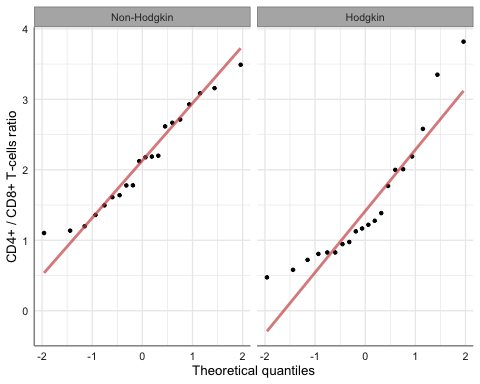Let’s say we choose a non-parametric test to compare the groups: wilcox.test(Ratio ~ Group, data = Hodgkin)  Wilcoxon rank sum exact test data: Ratio by Group W = 298, p-value = 0.007331 alternative hypothesis: true location shift is not equal to 0 ## 2.2 Graphical output For relatively small samples (for example, less than 30 observations per group) is a standard practice to show the actual data in dot plots with error bars. The pubh package offers two options to show graphically differences in continuous outcomes among groups: • For small samples: strip_error • For medium to large samples: bar_error For our current example: Hodgkin %>% strip_error(Ratio ~ Group) %>% axis_labs()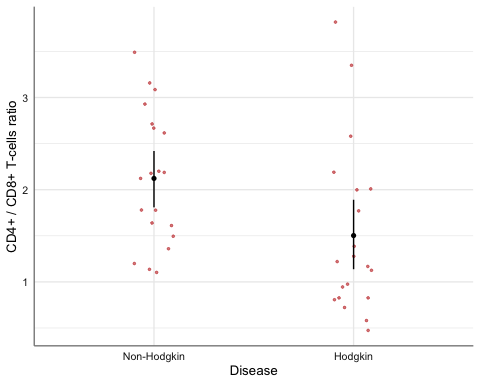In the previous code, axis_labs applies labels from labelled data to the axis. The error bars represent 95% confidence intervals around mean values. Is relatively easy to add a line on top, to show that the two groups are significantly different. The function gf_star needs the reference point on how to draw an horizontal line to display statistical difference or to annotate a plot; in summary, gf_star: • Draws an horizontal line from $$(x1, y2)$$ to $$(x2, y2)$$. • Draws a vertical line from $$(x1, y1)$$ to $$(x1, y1)$$. • Draws a vertical line from $$(x2, y1)$$ to $$(x2, y1)$$. • Writes a character (the legend or “star”) at the mid point between $$x1$$ and $$x2$$ at high $$y3$$. Thus: $y1 < y2 < y3$ In our current example: Hodgkin %>% strip_error(Ratio ~ Group) %>% axis_labs() %>% gf_star(x1 = 1, y1 = 4, x2 = 2, y2 = 4.05, y3 = 4.1, "**")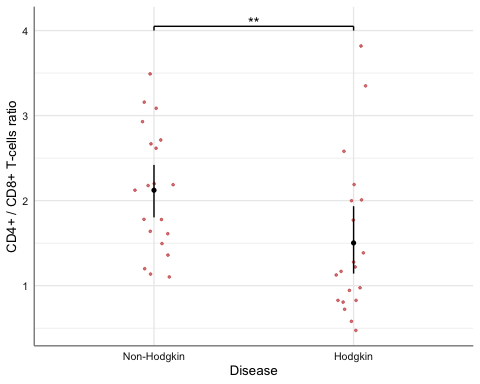For larger samples we could use bar charts with error bars. For example: data(birthwt, package = "MASS") birthwt <- birthwt %>% mutate( smoke = factor(smoke, labels = c("Non-smoker", "Smoker")), Race = factor(race > 1, labels = c("White", "Non-white")), race = factor(race, labels = c("White", "Afican American", "Other")) ) %>% var_labels( bwt = 'Birth weight (g)', smoke = 'Smoking status', race = 'Race', ) birthwt %>% bar_error(bwt ~ smoke) %>% axis_labs()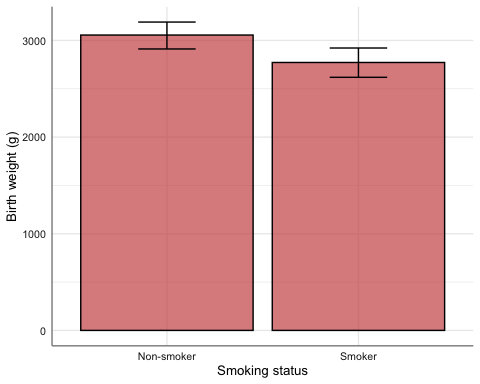Quick normality check: birthwt %>% qq_plot(~ bwt|smoke) %>% axis_labs()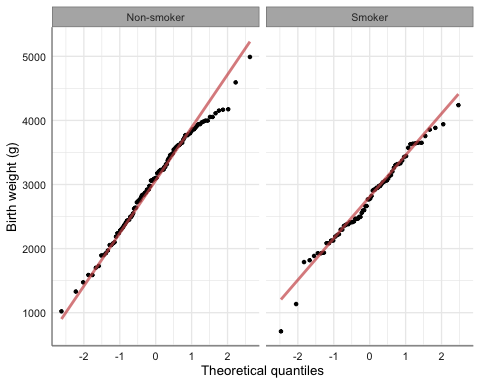The (unadjusted) $$t$$-test: t.test(bwt ~ smoke, data = birthwt)  Welch Two Sample t-test data: bwt by smoke t = 2.7299, df = 170.1, p-value = 0.007003 alternative hypothesis: true difference in means is not equal to 0 95 percent confidence interval: 78.57486 488.97860 sample estimates: mean in group Non-smoker mean in group Smoker 3055.696 2771.919  The final plot with annotation to highlight statistical difference (unadjusted): birthwt %>% bar_error(bwt ~ smoke) %>% axis_labs() %>% gf_star(x1 = 1, x2 = 2, y1 = 3400, y2 = 3500, y3 = 3550, "**")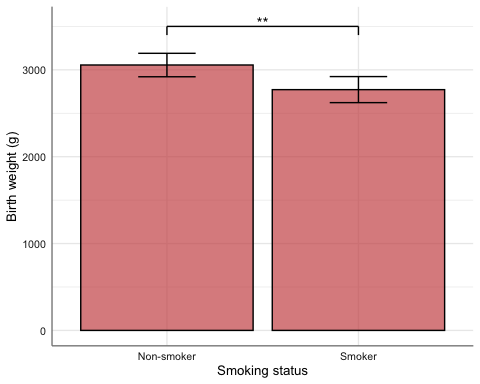Both strip_error and bar_error can generate plots stratified by a third variable, for example: birthwt %>% bar_error(bwt ~ smoke, fill = ~ Race) %>% gf_refine(ggsci::scale_fill_jama()) %>% axis_labs()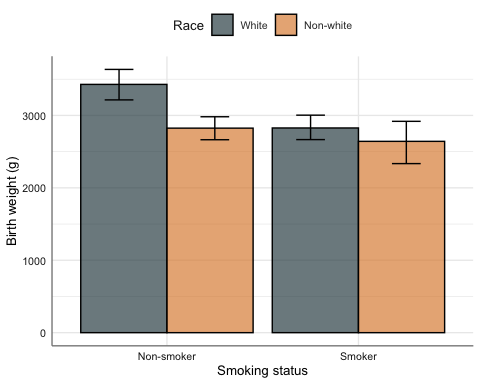birthwt %>% bar_error(bwt ~ smoke|Race) %>% axis_labs()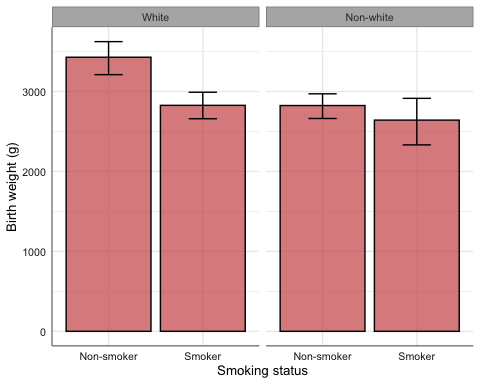birthwt %>% strip_error(bwt ~ smoke, pch = ~ Race, col = ~ Race) %>% gf_refine(ggsci::scale_color_jama()) %>% axis_labs()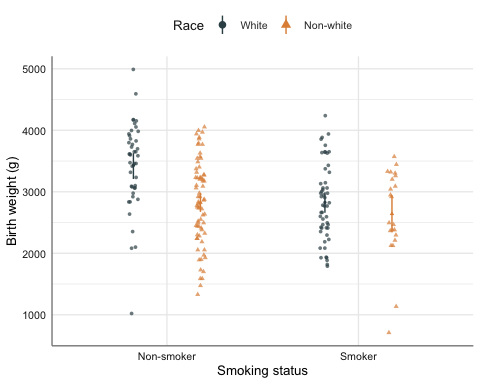# 3 Regression models The pubh package includes the function glm_coef for displaying coefficients from regression models and the function multiple to help in the visualisation of multiple comparisons. Note: Please read the vignette on Regression Examples for a more comprehensive use of glm_coef. For simplicity, here we show the analysis of the linear model of smoking on birth weight, adjusting by race (and not by other potential confounders). model_bwt <- lm(bwt ~ smoke + race, data = birthwt) model_bwt %>% glm_coef(labels = model_labels(model_bwt)) %>% as_hux() %>% set_align(everywhere, 2:3, "right") %>% add_footnote(get_r2(model_bwt), font_size = 9) ParameterCoefficientPr(>|t|) Constant3334.95 (3153.89, 3516.01)< 0.001 Smoking status: Smoker-428.73 (-643.86, -213.6)< 0.001 Race: Afican American-450.36 (-752.45, -148.27)0.004 Race: Other-452.88 (-682.67, -223.08)< 0.001 R2 = 0.123 Similar results can be obtained with the function tab_model from the sjPlot package. tab_model(model_bwt, collapse.ci = TRUE) Birth weight (g) Predictors Estimates p (Intercept) 3334.95 (3153.89 – 3516.01) <0.001 Smoking status: Smoker -428.73 (-643.86 – -213.60) <0.001 Race: Afican American -450.36 (-752.45 – -148.27) 0.004 Race: Other -452.88 (-682.67 – -223.08) <0.001 Observations 189 R2 / R2 adjusted 0.123 / 0.109 Some advantages of glm_coef over tab_model are: • Script documents can be knitted to Word and $$\LaTeX$$ (besides HTML). • Uses robust standard errors by default. The option to not use robust standard errors is part of the arguments. • Recognises some type of models that tab_model does not recognise. Some advantages of tab_model over glm_coef are: • Recognises labels from variables and use those labels to display the table. • Includes some statistics about the model. • It can display more than one model on the same output. • Tables are more aesthetically appealing. In the previous table of coefficients confidence intervals and p-values for race had not been adjusted for multiple comparisons. We use functions from the emmeans package to make the corrections. multiple(model_bwt, ~ race)df
                 contrast estimate     SE t.ratio p.value lower.CL upper.CL
1 Afican American - White  -450.36 153.12   -2.94    0.01  -810.84   -89.88
2           Other - White  -452.88 116.48   -3.89 < 0.001  -727.09  -178.66
3 Other - Afican American    -2.52 160.59   -0.02       1  -380.59   375.56
multiple(model_bwt, ~ race)$fig_ci %>% gf_labs(x = "Difference in birth weights (g)")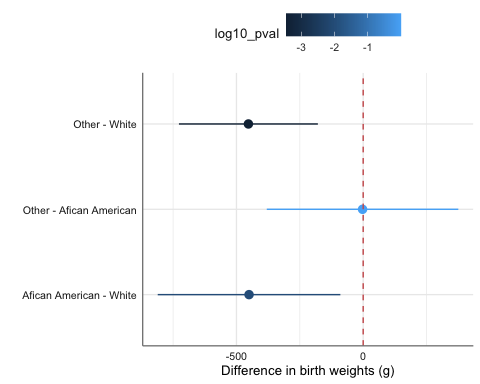multiple(model_bwt, ~ race)$fig_pval %>%
gf_labs(y = " ")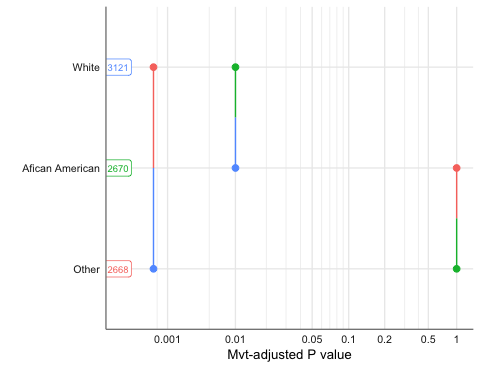# 4 Epidemiology functions

The pubh package offers two wrappers to epiR functions.

1. contingency calls epi.2by2 and it’s used to analyse two by two contingency tables.
2. diag_test calls epi.tests to compute statistics related with screening tests.

## 4.1 Contingency tables

Let’s say we want to look at the effect of ibuprofen on preventing death in patients with sepsis.

data(Bernard)
Bernard %>% head()
# A tibble: 6 x 9
id treat     race             fate  apache o2del followup temp0 temp10
<dbl> <fct>     <fct>            <fct>  <dbl> <dbl>    <dbl> <dbl>  <dbl>
1     1 Placebo   White            Dead      27  539.       50  35.2   36.6
2     2 Ibuprofen African American Alive     14   NA       720  38.7   37.6
3     3 Placebo   African American Dead      33  551.       33  38.3   NA
4     4 Ibuprofen White            Alive      3 1376.      720  38.3   36.4
5     5 Placebo   White            Alive      5   NA       720  38.6   37.6
6     6 Ibuprofen White            Alive     13 1523.      720  38.2   38.2

Let’s look at the table:

Bernard %>%
mutate(
fate = relevel(fate, ref = "Dead"),
treat = relevel(treat, ref = "Ibuprofen")
) %>%
copy_labels(Bernard) %>%
cross_tab(fate ~ treat) %>%
theme_pubh()
Mortality status
(N=176)(N=279)(N=455)
Treatment
- Ibuprofen84 (47.7%)140 (50.2%)224 (49.2%)
- Placebo 92 (52.3%)139 (49.8%)231 (50.8%)

For epi.2by2 we need to provide the table of counts in the correct order, so we would type something like:

dat <- matrix(c(84, 140 , 92, 139), nrow = 2, byrow = TRUE)
epiR::epi.2by2(as.table(dat))
             Outcome +    Outcome -      Total        Inc risk *        Odds
Exposed +           84          140        224              37.5       0.600
Exposed -           92          139        231              39.8       0.662
Total              176          279        455              38.7       0.631

Point estimates and 95% CIs:
-------------------------------------------------------------------
Inc risk ratio                               0.94 (0.75, 1.19)
Odds ratio                                   0.91 (0.62, 1.32)
Attrib risk *                                -2.33 (-11.27, 6.62)
Attrib risk in population *                  -1.15 (-8.88, 6.59)
Attrib fraction in exposed (%)               -6.20 (-33.90, 15.76)
Attrib fraction in population (%)            -2.96 (-15.01, 7.82)
-------------------------------------------------------------------
Test that OR = 1: chi2(1) = 0.260 Pr>chi2 = 0.61
Wald confidence limits
CI: confidence interval
* Outcomes per 100 population units 

For contingency we only need to provide the information in a formula:

Bernard %>%
contingency(fate ~ treat) 
           Outcome
Ibuprofen   84   140
Placebo     92   139

Outcome +    Outcome -      Total        Inc risk *        Odds
Exposed +           84          140        224              37.5       0.600
Exposed -           92          139        231              39.8       0.662
Total              176          279        455              38.7       0.631

Point estimates and 95% CIs:
-------------------------------------------------------------------
Inc risk ratio                               0.94 (0.75, 1.19)
Odds ratio                                   0.91 (0.62, 1.32)
Attrib risk *                                -2.33 (-11.27, 6.62)
Attrib risk in population *                  -1.15 (-8.88, 6.59)
Attrib fraction in exposed (%)               -6.20 (-33.90, 15.76)
Attrib fraction in population (%)            -2.96 (-15.01, 7.82)
-------------------------------------------------------------------
Test that OR = 1: chi2(1) = 0.260 Pr>chi2 = 0.61
Wald confidence limits
CI: confidence interval
* Outcomes per 100 population units

Pearson's Chi-squared test with Yates' continuity correction

data:  dat
X-squared = 0.17076, df = 1, p-value = 0.6794

Advantages of contingency:

1. Easier input without the need to create the table.
2. Displays the standard epidemiological table at the start of the output. This aids to check what are the reference levels on each category.
3. In the case that the $$\chi^2$$-test is not appropriate, contingency would show the results of the Fisher exact test at the end of the output.

### 4.1.1 Mantel-Haenszel odds ratio

For mhor the formula has the following syntax:

outcome ~ stratum/exposure, data = my_data

Thus, mhor displays the odds ratio of exposure yes against exposure no on outcome yes for different levels of stratum, as well as the Mantel-Haenszel pooled odds ratio.

Example: effect of eating chocolate ice cream on being ill by sex from the oswego data set.

data(oswego, package = "epitools")

oswego <- oswego %>%
mutate(
ill = factor(ill, labels = c("No", "Yes")),
sex = factor(sex, labels = c("Female", "Male")),
chocolate.ice.cream = factor(chocolate.ice.cream, labels = c("No", "Yes"))
) %>%
var_labels(
ill = "Developed illness",
sex = "Sex",
chocolate.ice.cream = "Consumed chocolate ice cream"
)

First we look at the cross-tabulation:

oswego %>%
mutate(
ill = relevel(ill, ref = "Yes"),
chocolate.ice.cream = relevel(chocolate.ice.cream, ref = "Yes")
) %>%
copy_labels(oswego) %>%
cross_tab(ill ~ sex + chocolate.ice.cream) %>%
theme_pubh()
Developed illness
YesNoTotal
(N=46)(N=29)(N=75)
Sex
- Female 30 (65.2%)14 (48.3%)44 (58.7%)
- Male 16 (34.8%)15 (51.7%)31 (41.3%)
Consumed chocolate ice cream
- Yes 25 (55.6%)22 (75.9%)47 (63.5%)
- No 20 (44.4%)7 (24.1%)27 (36.5%)
oswego %>%
mhor(ill ~ sex/chocolate.ice.cream)

OR Lower.CI Upper.CI Pr(>|z|)
sexFemale:chocolate.ice.creamYes 0.47     0.11     2.06    0.318
sexMale:chocolate.ice.creamYes   0.24     0.05     1.13    0.072

Common OR Lower CI Upper CI Pr(>|z|)
Cochran-Mantel-Haenszel:       0.35     0.12     1.01    0.085

Test for effect modification (interaction): p =  0.5434


### 4.1.2 Odds trend

Example: An international case-control study to test the hypothesis that breast cancer is related to the age that a woman gives childbirth.

data(Oncho)

odds_trend(mf ~ agegrp, data = Oncho, angle = 0, hjust = 0.5)\$fig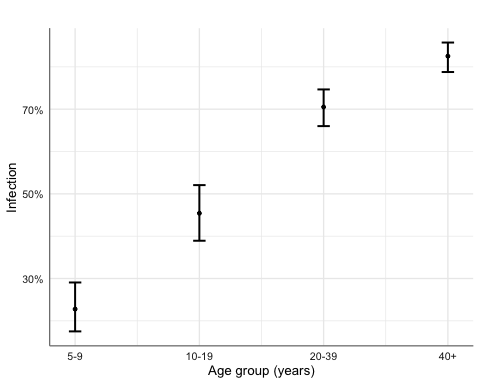## 4.2 Diagnostic tests

Example: We compare the use of lung’s X-rays on the screening of TB against the gold standard test.

Freq <- c(1739, 8, 51, 22)
BCG <- gl(2, 1, 4, labels=c("Negative", "Positive"))
Xray <- gl(2, 2, labels=c("Negative", "Positive"))
tb <- data.frame(Freq, BCG, Xray)
tb <- expand_df(tb)

diag_test(BCG ~ Xray, data = tb)
          Outcome +    Outcome -      Total
Test +           22           51         73
Test -            8         1739       1747
Total            30         1790       1820

Point estimates and 95 % CIs:
---------------------------------------------------------
Apparent prevalence                    0.04 (0.03, 0.05)
True prevalence                        0.02 (0.01, 0.02)
Sensitivity                            0.73 (0.54, 0.88)
Specificity                            0.97 (0.96, 0.98)
Positive predictive value              0.30 (0.20, 0.42)
Negative predictive value              1.00 (0.99, 1.00)
Positive likelihood ratio              25.74 (18.21, 36.38)
Negative likelihood ratio              0.27 (0.15, 0.50)
---------------------------------------------------------

Using the immediate version (direct input):

diag_test2(22, 51, 8, 1739)

Outcome +    Outcome -      Total
Test +           22           51         73
Test -            8         1739       1747
Total            30         1790       1820

Point estimates and 95 % CIs:
---------------------------------------------------------
Apparent prevalence                    0.04 (0.03, 0.05)
True prevalence                        0.02 (0.01, 0.02)
Sensitivity                            0.73 (0.54, 0.88)
Specificity                            0.97 (0.96, 0.98)
Positive predictive value              0.30 (0.20, 0.42)
Negative predictive value              1.00 (0.99, 1.00)
Positive likelihood ratio              25.74 (18.21, 36.38)
Negative likelihood ratio              0.27 (0.15, 0.50)
---------------------------------------------------------